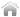HOW-TO:Use SMS-style text entry for remotesRemote controlsHOW-TO:Use SMS-style text entry for remotes

If you have a remote control that is viewed as a keyboard to Kodi and want to enable SMS-style text input and page jumping, this guide wills how you how to enable this using a custom keymap. If you have a normal "MCE" style remote or non-Android CEC device, then this how-to may not necessary.

1 Steps

1 Create a plain text file (no rich text formatting, don't use .doc, etc) and save it as keyboard.xml. Make sure that the file extension is ".xml" and not ".txt" or ".xml.txt".
2 Cut and paste this into your new plain text file:
```<?xml version="1.0" encoding="UTF-8"?>
<keymap>
<global>
<keyboard>
<zero>Number0</zero>
<one>JumpSMS1</one>
<two>JumpSMS2</two>
<three>JumpSMS3</three>
<four>JumpSMS4</four>
<five>JumpSMS5</five>
<six>JumpSMS6</six>
<seven>JumpSMS7</seven>
<eight>JumpSMS8</eight>
<nine>JumpSMS9</nine>
<escape>Back</escape>
</keyboard>
</global>
<VirtualKeyboard>
<keyboard>
<zero>Number0</zero>
<one>Number1</one>
<two>Number2</two>
<three>Number3</three>
<four>Number4</four>
<five>Number5</five>
<six>Number6</six>
<seven>Number7</seven>
<eight>Number8</eight>
<nine>Number9</nine>
<backspace>Backspace</backspace>
</keyboard>
</VirtualKeyboard>
<FullscreenVideo>
<keyboard>
<zero>Number0</zero>
<one>Number1</one>
<two>Number2</two>
<three>Number3</three>
<four>Number4</four>
<five>Number5</five>
<six>Number6</six>
<seven>Number7</seven>
<eight>Number8</eight>
<nine>Number9</nine>
<escape>Stop</escape>
</keyboard>
</FullscreenVideo>
<keyboard>
<zero>Number0</zero>
<one>Number1</one>
<two>Number2</two>
<three>Number3</three>
<four>Number4</four>
<five>Number5</five>
<six>Number6</six>
<seven>Number7</seven>
<eight>Number8</eight>
<nine>Number9</nine>
</keyboard>
<NumericInput>
<keyboard>
<zero>Number0</zero>
<one>Number1</one>
<two>Number2</two>
<three>Number3</three>
<four>Number4</four>
<five>Number5</five>
<six>Number6</six>
<seven>Number7</seven>
<eight>Number8</eight>
<nine>Number9</nine>
</keyboard>
</NumericInput>
<Teletext>
<keyboard>
<zero>number0</zero>
<one>number1</one>
<two>number2</two>
<three>number3</three>
<four>number4</four>
<five>number5</five>
<six>number6</six>
<seven>number7</seven>
<eight>number8</eight>
<nine>number9</nine>
</keyboard>
</Teletext>
</keymap>
```
3 Save this file in userdata/Keymaps/:

Note: if you use the Keymap Editor addon for Kodi, you need to append these new XML lines above inside the XML file generated by this addon, not in keyboard.xml. In March of 2015, the file generated by Keymap Editor is called "gen.xml".

4 If Kodi is already running then restart it so that the changes take effect.
5 Note: This step is not required for Kodi v14 and higher.

Go into Kodi and then Settings -> System -> Input devices and enable Remote control sends keyboard presses. This will allow your arrow keys to use the on-screen keyboard even though Kodi thinks your remote is a keyboard already.